# GameBoy Opcode Summary

The GameBoy has instructions & registers similiar to the 8080, 8085, & Z80 microprocessors. The internal 8-bit registers are A, B, C, D, E, F, H, & L. Theses registers may be used in pairs for 16-bit operations as AF, BC, DE, & HL. The two remaining 16-bit registers are the program counter (PC) and the stack pointer (SP).

The F register holds the cpu flags. The operation of these flags is identical to their Z80 relative. The lower four bits of this register always read zero even if written with a one.

 Flag Register 7 6 5 4 3 2 1 0 Z N H C 0 0 0 0

The GameBoy CPU is based on a subset of the Z80 microprocessor. A summary of these commands is given below.

 Mnemonic Symbolic Operation Comments CPU Clocks Flags - Z,N,H,C

 LD r,s rs s=r,n,(HL) r=4, n=8, (HL)=8 LD d,r dr d=r,(HL) r=4, (HL)=8 LD d,n dn r=8, (HL)=12 LD A,(ss) A(ss) ss=BC,DE,HL,nn [BC,DE,HL]=8, nn=16 LD (dd),A (dd)A dd=BC,DE,HL,nn LD A,(C) A(\$FF00+C) - 8 LD (C),A (\$FF00+C)A 8 LDD A,(HL) A(HL), HLHL - 1 8 LDD (HL),A (HL)A, HLHL - 1 8 LDI A,(HL) A(HL), HLHL + 1 8 LDI (HL),A (HL)A, HLHL + 1 8 LDH (n),A (\$FF00+n)A 12 LDH A,(n) A(\$FF00+n) 12

 LD dd,nn ddnn dd=BC,DE,HL,SP 12 - - - - LD (nn),SP (nn)SP - 20 LD SP,HL SPHL 8 LD HL,(SP+e) HL(SP+e) 12 0 0 * * PUSH ss (SP-1)ssh, (SP-2)ssl, SPSP-2 ss=BC,DE,HL,AF 16 - - - - POP dd ddl(SP), ddh(SP+1), SPSP+2 dd=BC,DE,HL,AF 12

### 8-Bit ALU

 ADD A,s AA + s CY is the carry flag. s=r,n,(HL) r=4, n=8, (HL)=8 * 0 * * ADC A,s AA + s + CY SUB s AA - s * 1 * * SBC A,s AA - s - CY AND s AA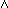s * 0 1 0 OR s AA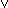s * 0 0 0 XOR s AAs CP s A - s * 1 * * INC s ss + 1 s=r,(HL) r=4, (HL)=12 * 0 * - DEC s ss - 1 * 1 * -

### 16-Bit Arithmetic

 ADD HL,ss HLHL + ss ss=BC,DE,HL,SP 8 - 0 * * ADD SP,e SPSP + e 16 0 0 * * INC ss ssss + 1 8 - - - - DEC ss ssss - 1 8

### Miscellaneous

 SWAP s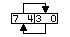Swap nibbles. s=r,(HL) r=8, (HL)=16 * 0 0 0 DAA Converts A into packed BCD. - 4 * - 0 * CPL A/A 4 - 1 1 - CCF CY/CY CY is the carry flag. 4 - 0 0 * SCF CY1 4 - 0 0 1 NOP No operation. - 4 - - - - HALT Halt CPU until an interrupt occurs. 4 STOP Halt CPU. 4 DI Disable Interrupts. 4 EI Enable Interrupts. 4

### Rotates & Shifts

 RLCA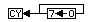4 0 0 0 * RLA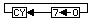RRCA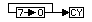RRA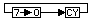RLC ss=A,r,(HL) r=8,(HL)=16 * 0 0 * RL sRRC sRR sSLA s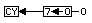s=r,(HL) r=8, (HL)=16 SRA s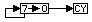SRL s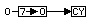### Bit Opcodes

 BIT b,s Z/sb Z is zero flag. s=r,(HL) r=8, (HL)=12 * 0 1 - SET b,s sb1 r=8, (HL)=16 - - - - RES b,s sb0

### Jumps

 JP nn PCnn - 16 JP cc,nn If cc is true, PCnn, else continue. If cc is true, 16 else 12. JP (HL) PCHL 4 JR e PCPC + e 12 JR cc,e if cc is true, PCPC + e, else continue. If cc is true, 12 else 8.

### Calls

 CALL nn (SP-1)PCh, (SP-2)PCl, PCnn, SPSP-2 - 24 CALL cc,nn If condition cc is false continue, else same as CALL nn. If cc is true, 24 else 12.

### Restarts

 RST f (SP-1)PCh, (SP-2)PCl, PCh0, PClf, SPSP-2 - 16

### Returns

 RET pcl(SP), pch(SP+1), SPSP+2 - 16 RET cc If cc is true, RET else continue. If cc is true, 20 else 8. RETI Return then enable interrupts. 16

### Terminology

 - Flag is not affected by this operation. * Flag is affected according to result of operation. b A bit number in any 8-bit register or memory location. C Carry flag. cc Flag condition code: C,NC,NZ,Z d Any 8-bit destination register or memory location. dd Any 16-bit destination register or memory location. e 8-bit signed 2's complement displacement. f 8 special call locations in page zero. H Half-carry flag. N Subtraction flag. NC Not carry flag NZ Not zero flag. n Any 8-bit binary number. nn Any 16-bit binary number. r Any 8-bit register. (A,B,C,D,E,H, or L) s Any 8-bit source register or memory location. sb A bit in a specific 8-bit register or memory location. ss Any 16-bit source register or memory location. Z Zero Flag.